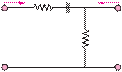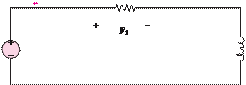### Create an Account

Home / Questions / In the circuit shown in Figure P611 determine the frequency response function in the form ...

# In the circuit shown in Figure P611 determine the frequency response function in the form b An expression for the voltage transfer function and show that it can be manipulated into the form

In the circuit shown in Figure P6.11, determine the frequency response function in the form

b.    An expression for the voltage transfer function and show that it can be manipulated into the form

H  jω)      Vo( jω)v             =  V ( jω)  = 1

i

Hvo

± jf (ω)

Hvjω) = 1

Hojf (ω)

and plot Hv( jω).Ii(w)                                        Io(w)

+           R1        C               +

I                              R+

Vjw)

R2       Vo   jw)

 V

 2

S                                                                                     V

Jun 18 2020 View more View LessSubscribe To Get Solution